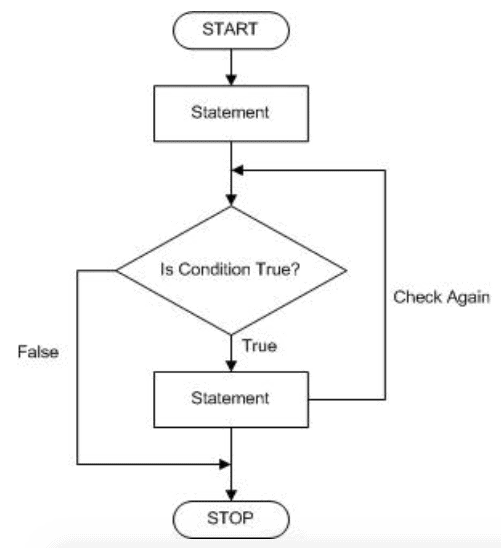# >>>for <var> in range <infinite>: ?

So we've learned about how to make a loop for a certain number of times, but is there a way to make a loop go on forever? Like if I wanted to run a code that constantly generates random numbers for the rest of time, would I be able to do that with Python?

Or, if I wanted to know how long it would take an equation to reach a certain number with different inputs but I don't know how many times it would take, how could I express that in Python?

+3

Yesss you can do that
We will learn that later on in the course.
Typically when you use a while loop it will keep on running until you stop the execution of the code

+1

Umer is right. We will learn this later in the course.

I know some of you feel like you've had a lot of different code commands introduced quickly already. And you can actually do a lot with just FOR loops!

The answer is Yes, Python could express an infinite loop.
But instead of using for i in range() loop, we will use while loop within a set of conditions.

The basic form of while() syntax is

``````while <condition>:
<body>
``````

Below is a flowchart of a while loop:
* `<statement>` in this pic is similar to `<body>`Explanation:
The way that while() syntax runs based on the condition.
If the condition is true, the body will execute repeatedly many times, even infinitely.
If the condition is false, the body will not execute at all.

An example of running infinitely:

``````i = 0
While i<3:
print('Using while loop')
``````

Since your initial input is 0 which is always less than 3, Python will print the text "Using while loop" for thousand times, even infinitely.

Another similar example that the condition is false, which results in terminating the loop:

``````i = 0
While i<3:
print('Using while loop')
i = i + 1
``````

Now, i = i + 1 here is to count i after finishing each loop.

• First loop: i = 0 => meet the condition i<3 **=>** then print the text
After first loop, the value of i is counted by adding 1 => the value of i becomes 1 ( 0 + 1 = 1)

• Second loop: i = 1 => meet the condition i<3 **=>** then print the text
After second loop, the current value of i is counted by adding 1 => the value of i becomes 1 ( 1 + 1 = 2)

• Third loop: i = 2 => meet the condition i<3 **=>** then print the text.
After third loop, the current value of i is counted by adding 1 => the value of i becomes 1 ( 2 + 1 = 3)

• Fourth loop: i = 3 => not meet the condition i<3 **=> not** print the text.
In the fourth loop, Python will stop this loop since it doesn't meet the condition anymore.

The second example could be expressed under the range() syntax like:

``````for i in range(3)
print('Using while loop')
``````

That's how a while loop works for the purpose of creating an infinite loop. Acc, Im not good at using while loop, so Idk how to explain this one for you to understand best. I think that we will learn abt this while loop soon in this CSC class (im not sure but hope so).

Hope this helps anyway!